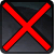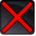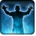# [passive]Conditions

## Used by

Used by
• Class 16140980780850385800

## Related effects, buffs and debuffs

Please click on an effect below to view its details.

• [hidden] [does not expire]Effect #1

 Slot: Buff Duration: passive Tick rate: does not tick # occurrences: 0
• On Apply

Perform the following actions:

• Modify stat Max Health, set to 365.97886%
• Play appearance epp . qtr . 1x6 . raid . darvannis . trash . secret . hateful_appearance
• When an ability is activated by NONE

Perform the following actions:

• Add effect #3 to TARGET from TARGET
• Add effect #4 to TARGET from TARGET
• [does not expire]Ancient Slumber
Each stack of Ancient Slumber reduces damage taken by 10%.

 Slot: Buff Duration: passive Tick rate: does not tick Initial stacks: 8 Max stacks: 8 # occurrences: 0 Tags: tag.​abl.​qtr.​1x4.​raid.​asation.​enemy.​trash.​secret.​dreadful_slumber
• When effect is applied

Perform the following actions:

• When stacks of this effect decrease

Only when the following conditions are met:

• If stacks of this effect = 5

Perform the following actions:

• When stacks of this effect decrease

Only when the following conditions are met:

• If stacks of this effect = 4

Perform the following actions:

• When stacks of this effect decrease

Only when the following conditions are met:

• If stacks of this effect = 3

Perform the following actions:

• When stacks of this effect decrease

Only when the following conditions are met:

• If stacks of this effect = 2

Perform the following actions:

• When stacks of this effect decrease

Only when the following conditions are met:

• If stacks of this effect = 1

Perform the following actions:

• When stacks of this effect decrease

Only when the following conditions are met:

• If stacks of this effect = 0

Perform the following actions:

• [hidden] [does not expire]Effect 3242975186387214

 Slot: Buff Duration: passive Tick rate: does not tick Initial stacks: 1 Max stacks: 10 # occurrences: 1 Conditions: Can only by called by other effects
• When stacks of this effect increase
• When effect is applied

Only when the following conditions are met:

• If stacks of this effect = 1

Perform the following actions:

• Modify meta stat #62, fixed = -0.2
• When stacks of this effect increase

Only when the following conditions are met:

• If stacks of this effect = 2

Perform the following actions:

• Modify meta stat #62, fixed = -0.4
• When stacks of this effect increase

Only when the following conditions are met:

• If stacks of this effect = 3

Perform the following actions:

• Modify meta stat #62, fixed = -0.6
• When stacks of this effect increase

Only when the following conditions are met:

• If stacks of this effect = 4

Perform the following actions:

• Modify meta stat #62, fixed = -0.8
• When stacks of this effect increase

Only when the following conditions are met:

• If stacks of this effect = 5

Perform the following actions:

• Modify meta stat #62, fixed = -1
• When stacks of this effect increase

Only when the following conditions are met:

• If stacks of this effect = 6

Perform the following actions:

• Modify meta stat #62, fixed = -1.2
• When stacks of this effect increase

Only when the following conditions are met:

• If stacks of this effect = 7

Perform the following actions:

• Modify meta stat #62, fixed = -1.4
• When stacks of this effect increase

Only when the following conditions are met:

• If stacks of this effect = 8

Perform the following actions:

• Modify meta stat #62, fixed = -1.6
• When stacks of this effect increase

Only when the following conditions are met:

• If stacks of this effect = 9

Perform the following actions:

• Modify meta stat #62, fixed = -1.8
• When stacks of this effect increase

Only when the following conditions are met:

• If stacks of this effect = 10

Perform the following actions:

• Modify meta stat #62, fixed = -2
• [hidden] [3s]Effect #4

 Slot: Buff Duration: 3s Tick rate: does not tick # occurrences: 0 Conditions: Can only by called by other effects
• When effect expires

Perform the following actions:

• Add effect #5 to TARGET from TARGET
• [hidden] [30s]Effect #5

 Slot: Buff Duration: 30s Tick rate: does not tick # occurrences: 0 Tags: tag.​abl.​qtr.​1x4.​raid.​asation.​enemy.​trash.​secret.​awakening_gate Conditions: Can only by called by other effects